## Be a geoscience and data science detective

### Featured

September 16, 2020

#### Introduction

These days everyone talks about data science. But here’s a question: if you are a geoscientist, and like me you have some interest in data science (that is, doing more quantitative and statistical analyses with data), why choose between the two? Do both… always! Your domain knowledge is an indispensable condition, and so is an attitude of active curiosity (for me, an even more important condition). So, my advice for aspiring geoscientists and data scientists is: “yes, do some course work, read some articles, maybe a book, get the basics of Python or R, if you do not know a programming language” but then jump right ahead into doing! But – please! – skip the Titanic, Iris, Cars datasets, or any other data from your MOOC or that many have already looked at! Get some data that is interesting to you as geoscientist, or put it together yourself.

#### First example: evaluate the quality of seismic inversion from a published article

In this first example, the one presented at Transform 2020, I wanted to evaluate the quality of seismic inversion from a published article in the November 2009 CSEG Recorder Inversion Driven Processing. In the article, the evaluation was done by the authors at a blind well location, but only qualitatively, as illustrated in Figure 5, shown below for reference. In the top panel (a) the evaluation is for the inversion without additional processing (SPNA, Signal Protected Noise Attenuation); in the bottom panel (b) the evaluation is for the inversion with SPNA. On the right side of each panel the inverted seismic trace is plotted against the upscaled impedance well log (calculated by multiplying the well density log and the well velocity log from compressional sonic); on the right, the upscaled impedance log is inserted in a seismic impedance section as a colored trace (at the location of the well) using the same color scale and range used for the impedance section.Figure 5 caption: Acoustic impedance results at the blind well for data without (a) and with (b) SPNA. The figure shows a 200 ms window of inverted seismic data with well B, the blind well, in the middle on the left, along with acoustic impedance curves for the well (red) and inverted seismic (blue) on the right. The data with SPNA shows a better fit to the well, particularly over the low frequencies.

What the authors reported in the figure caption is the extent to which the evaluation was discussed in the paper; unfortunately it is not backed up in any quantitative way, for example comparing a score, such as R^2, for the two methods. Please notice that I am not picking on this paper in particular, which in fact I rather quite liked, but I am critical of the lack of supporting statistics, and wanted to supplement the paper with my own.

In order to do that, I hand-digitized from the figure above the logs and inversion traces , then interpolated to regularly-sampled time intervals (by the way: if you are interested in a free tool to  digitize plots, check  use WebPlotDigitizer).

My plan was to split my evaluation in an upper and lower zone, but rather than using the seismically-picked horizon, I decided to add a fake top at 1.715 seconds, where I see a sharp increase in impedance in Figure 5. This was an arbitrary choice on my part of a more geological horizon, separating the yellow-red band from the green blue band in the impedance sections. The figure below shows all the data in a Matplotlib figure:The first thing I did then, was to look at the Root Mean Square Error in the upper and lower zone obtained using the fake top. They are summarized in the table below: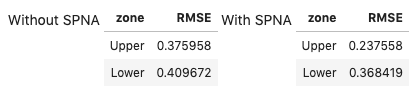Based on the RMSE , it looks like case b, the inversion with Signal Protected Noise Attenuated applied on the data, is a better result for the Upper zone, but not for the Lower one. This result is in agreement with my visual comparison of the two methods.

But lets’ dig a bit deeper. After looking at RMSE, I used the updated version of the error_flag function, which I first wrote at the 2018 Geophysics Sprint, listed below:

def error_flag(pred, actual, stat, dev = 1.0, method = 1):
"""Calculate the difference between a predicted and an actual curve
and return a curve flagging large differences based on a user-defined distance
(in deviation units) from either the mean difference or the median difference

Matteo Niccoli, October 2018. Updated in May 2020.

Parameters:
predicted : array
predicted log array
actual : array
original log array
stat : {‘mean’, ‘median’}
The statistics to use. The following options are available:
- mean: uses numpy.mean for the statistic,
and np.std for dev calculation
- median: uses numpy.median for the statistic,
and scipy.stats.median_absolute_deviation (MAD) for dev calculation
dev : float, optional
the standard deviations to use. The default is 1.0
method : int {1, 2, 3}, optional
The error method to use. The following options are available
(default is 1):
1: difference between curves larger than mean difference plus dev
2: curve slopes have opposite sign (after a 3-sample window smoothing)
3: curve slopes of opposite sign OR difference larger than mean plus dev
Returns:
flag : array
The error flag array
"""

flag = np.zeros(len(pred))
err = np.abs(pred-actual)

if stat == 'mean':
err_stat = np.mean(err)
err_dev = np.std(err)
elif stat == 'median':
err_stat = np.median(err)
err_dev = sp.stats.median_absolute_deviation(err)

pred_sm = pd.Series(np.convolve(pred, np.ones(3), 'same'))
actual_sm = pd.Series(np.convolve(actual, np.ones(3), 'same'))
ss = np.sign(pred_sm.diff().fillna(pred_sm))
ls = np.sign(actual_sm.diff().fillna(actual_sm))

if method == 1:
flag[np.where(err>(err_stat + (dev*err_dev)))] = 1
elif method == 2:
flag[np.where((ss + ls)==0 )]= 1
elif method == 3:
flag[np.where(np.logical_or(err>(err_stat + (dev*err_dev)), (ss+ls)==0 ))]= 1
return flag

I believe this new version is greatly improved because:

• Users now can choose between mean/standard deviation and median/median absolute deviation as a statistic for the error. The latter is more robust in the presence of outliers
• I added a convolutional smoother prior to the slope calculation, so as to make it less sensitive to noisy samples
• I expanded and improved the doctstring

The figure below uses the flag returned by the function to highlight areas of poorer inversion results, which I assigned based on passed to the function very restrictive parameters:

• using median and a median absolute deviation of 0.5 to trigger flag
• combining the above with checking for the slope signI also wrote short routines to count the number and percentage of samples that have been flagged, for each result, which are summarized in the table below: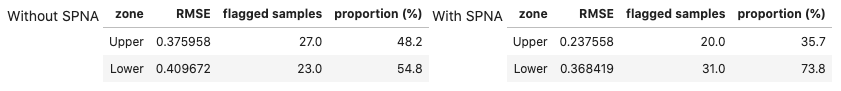The error flag method is in agreement with the RMS result: case b, the inversion on Signal Protected Noise Attenuated data is a better result for the Upper zone, but for the Lower zone the inversion without SPNA is the better one. Very cool!

But I was not satisfied yet. I was inspired to probe even deeper after a number of conversations with my friend Thomas Speidel, and reading the chapter on Estimation from Computational and Inferential Thinking (UC Berkeley). Specifically, I was left with the question in mind: “Can we be confident about those two is better in the same way?

This question can be answered with a bootstrapped Confidence Interval for the proportions of flagged samples, which I do below using the code in the book, with some modifications and some other tools from the datascience library. The results are shown below. The two plots, one for the Upper and one for the Lower zone, show the distribution of bootstrap flagged proportions for the two inversion results, with SPNA in yellow, and without SPNA in blue, respectively, and the Confidence Intervals in cyan and brown, respectively (the CI upper and lower bounds are also added to the table, for convenience).

By comparing the amount (or paucity) of overlap between the distributions (and between the confidence intervals) in the two plots, I believe I can be more confident in the conclusion drawn for the Lower zone, which is that the inversion on data without SPNA is better (less proportion of flagged errors), as there is far less overlap.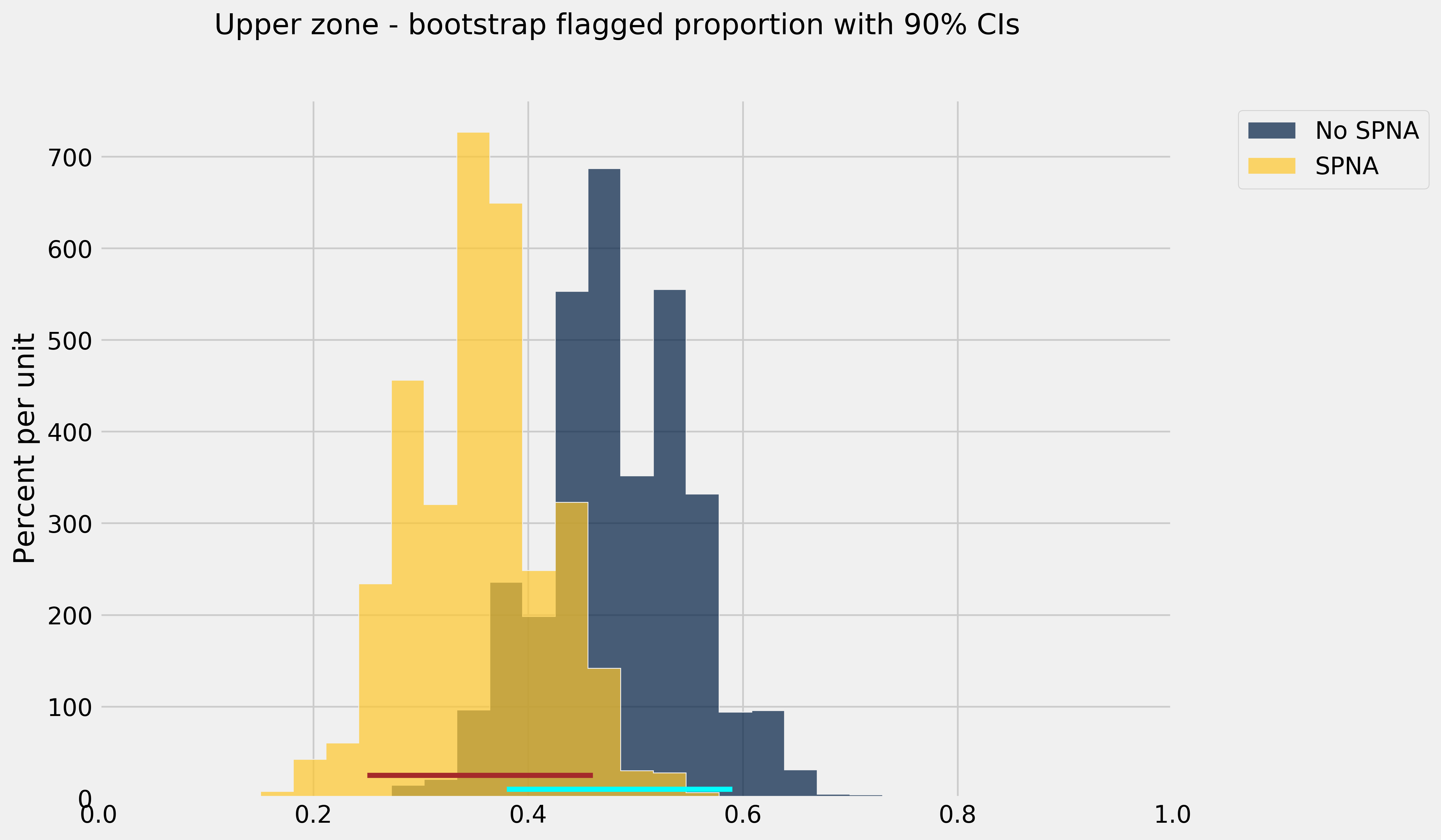I am very pleased with these results. Of course, there are some caveats to keep in mind, mainly that:
• I may have introduced small, but perhaps significant errors with hand digitizing
• I chose a statistical measure (the median and median absolute deviation) over a number of possible ones
• I chose an arbitrary geologic reference horizon without a better understanding of the reservoir and the data, just the knowledge from the figure in the paper

However, I am satisfied with this work, because it points to a methodology that I can use in the future. And I am happy to share it! The Jupyter notebook is available on GitHub.

#### Second example: evaluate regression results from a published article

My second, more recent example, is shorter but no less interesting, in my opinion. It is a case study correlating inversion data to petrophysical estimates of porosity-height in the Doig and Montney Formations in Western Canada (from the paper Tight gas geophysics: AVO inversion for reservoir characterization, Close et al. CSEG Recorder, May 2010, an article which I enjoyed very much reading).

The authors indicated that Vp/Vs and/or Poisson’s ratio maps from seismic inversion are good indicators of porosity in the Lower Doig and Upper Montney reservoirs in the wells used in their study, so it was reasonable to try to predict Phi-H from Vp/Vs via regression. The figure below, from the article, shows one such Vp/Vs ratio map and the Vp/Vs vs. Phi-H cross-plot for 8 wells.Figure 7 caption: Figure 7. a) Map of median Vp/Vs ratio value and porosity-height from 8 wells through the Lower Doig and Upper Montney. The red arrows highlight wells with very small porosity-height values and correspond in general to areas of higher Vp/Vs ratio. The blue arrow highlights a well at the edge of the seismic data where the inversion is adversely affected by decreased fold. The yellow line is the approximate location of a horizontal well where micro-seismic data were recorded during stimulation. b) Cross-plot of porosity-height values as shown in (a) against Vp/Vs extracted from the map. The correlation co-efficient of all data points (blue line) of 0.7 is improved to 0.9 (red line) by removing the data point marked by the blue arrow which again corresponds to the well near the edge of the survey (a).

They also show in the figure that by removing one of the data points, corresponding to a well near the edge of the survey (where the seismic inversion result is presumably not as reliable, due to lower offset and azimuth coverage), the correlation co-efficient is improved from 0.7 to 0.9 (red line).

So, the first thing I set out to do was to reproduce the crossplot.  I again hand-digitized the porosity-height and Vp/Vs pairs in the cross-plot using again WebPlotDigitizer. However, switched around the axes, which seems more natural to me since the objectives of regression efforts would be to predict as the dependent variable Phi-h, at the location of yet to drill wells, given Vp/Vs from seismic inversion. I also labelled the wells using their row index, after having loaded them in a Pandas DataFrame. And I used Ordinary Least Square Regression from the statsmodels library twice: once with all data points, the second time after removal of the well labelled as 5 in my plot above.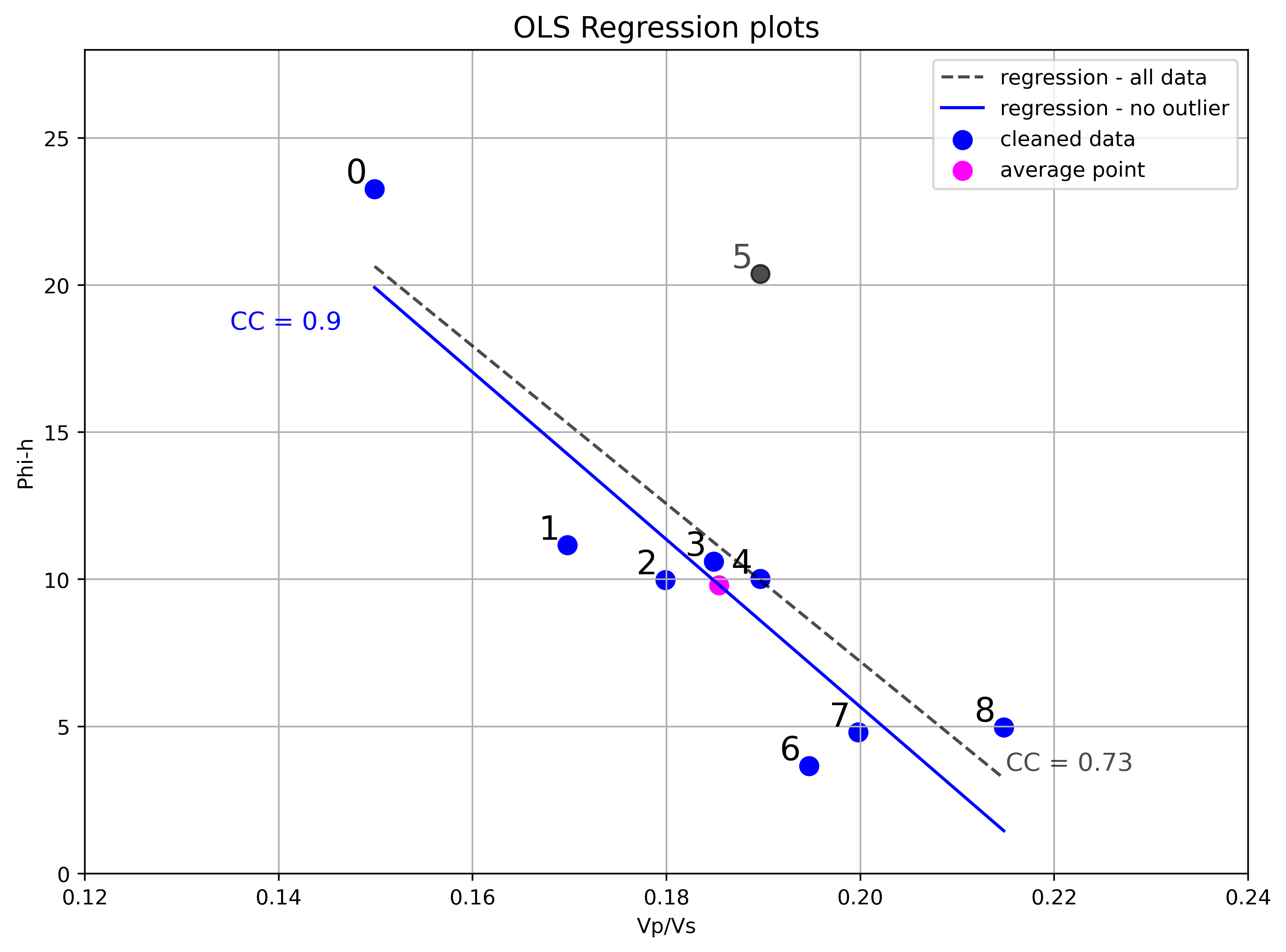So, I am able to reproduced the analysis from the figure. I think removing an outlier with insight from domain knowledge (the observation that poorer inversion result at this location is reasonable for the deviation from trend) is a legitimate choice. However, I would like to dig a bit deeper, to back up the decision with other analyses and tests, and to show how one might do it with their own data.

The first thing to look at is an Influence plot, which is a plot of the residuals, scaled by their standard deviation, against the leverage, for each observation. Influence plots are useful to distinguish between high leverage observations from outliers and are one of statsmodel ‘s standard Regression plots, so we get the next figure almost for free, with minor modifications to the default example). Here it is below, together with the OLS regression result, with all data points.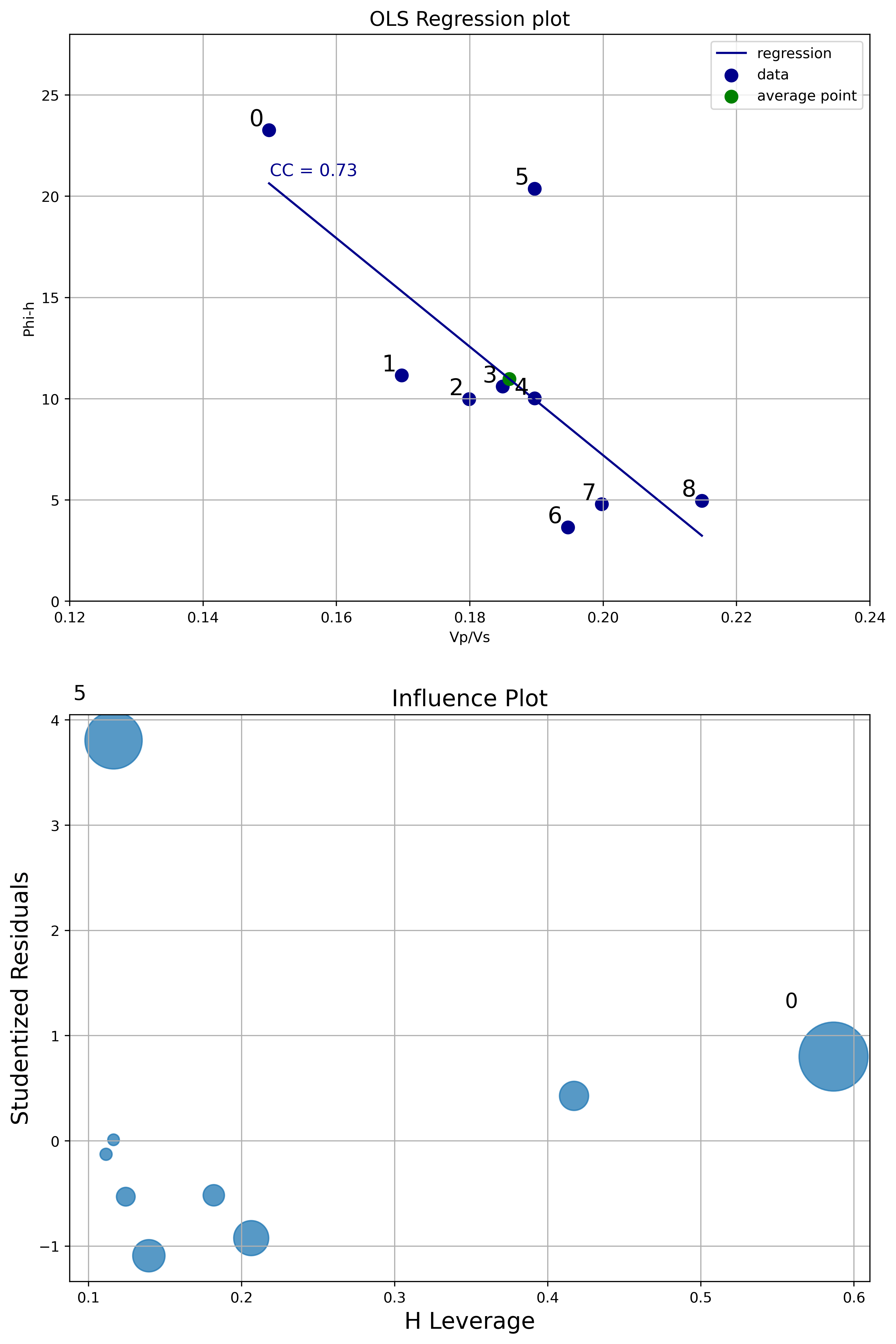From the Influence plot it is very obvious that the point labelled as zero has high leverage (but not high normalized residual). This is not a concern because points with high leverage are important but do not alter much the regression model. On the other hand, the point labelled as 5 has very high normalized residual. This point is an outlier and it will influence the regression line, reducing the R^2 and correlation coefficient. This analysis is a robust way to legitimize removing that data point.

Next I run some inferential tests. As I’ve written in an earlier notebook on Data loading, visualization, significance testing, I find the critical r very useful in the context of bivariate analysis. The critical r is the value of the correlation coefficient at which you can rule out chance as an explanation for the relationship between variables observed in the sample, and I look at it in combination with the confidence interval of the correlation coefficient.

The two plots below display, in dark blue and light blue respectively, the upper and lower confidence interval bounds, as the correlation coefficient r varies between 0 and 1 (x axis). These two bounds will change with different number of wells (they will get closer with more wells, and farther apart with less wells). The lower bound intersects the x axis (y=0) axis at a value equal to the critical r (white dot). The green dots highlight the actual confidence interval for a specific correlation coefficient chosen, in this case 0.73 with 9 wells, and 0.9 with 8 wells.

By the way: these plots are screen captures from the interactive tool I built taking advantage of the Jupyter interactive functionality (ipywidgets). You can try the tool by running the Jupyter notebook.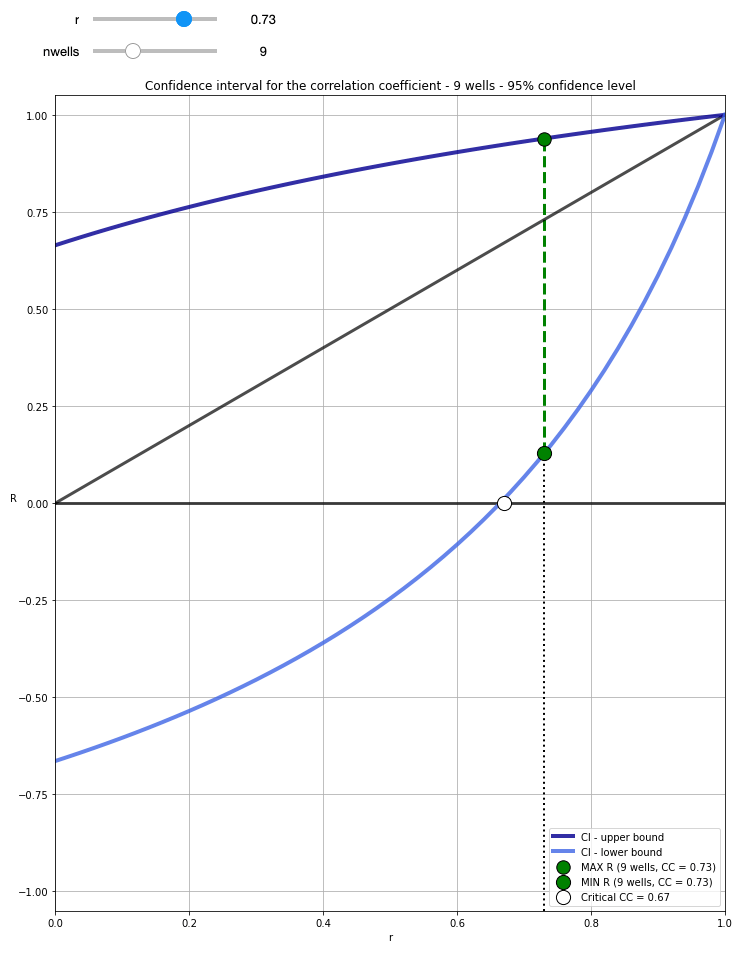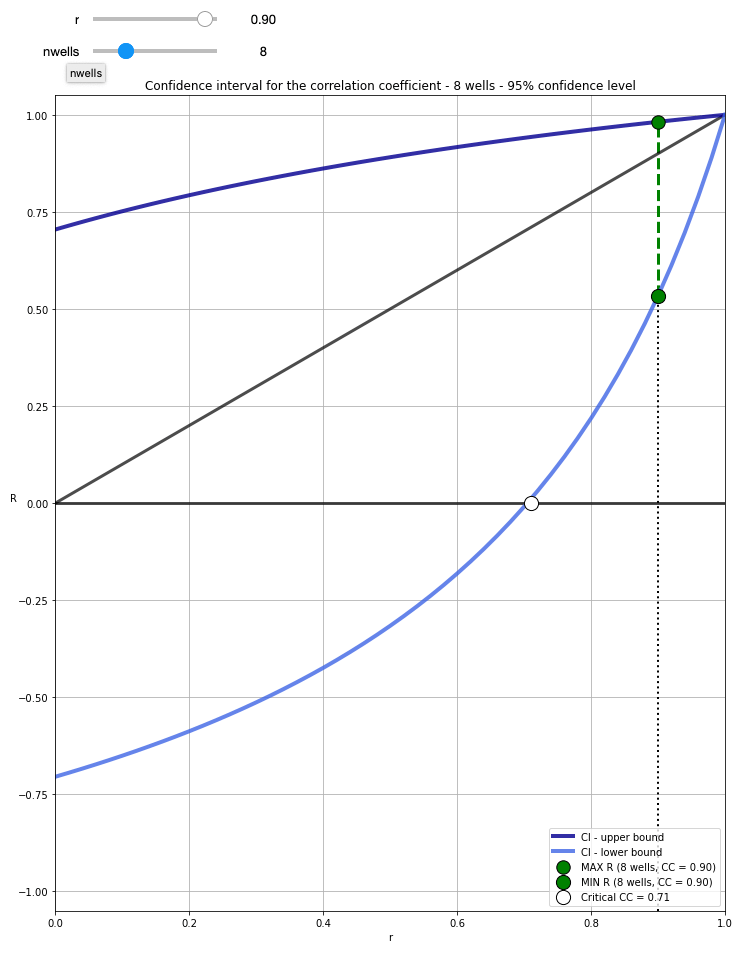With 9 wells, and cc=0.73, the resulting critical r = 0.67 tells us that for a 95% confidence level (0.05 alpha) we need at least a correlation coefficient of 0.67 in the sample (the 9 wells drilled) to be able to confidently say that there is correlation in the population (e.g. any well, future wells). However, the confidence interval is quite broad, ranging between 0.13 and 0.94 (you can get these numbers by running confInt(0.73, 9) in a cell.

With 8 wells (having removed the outlier), CC=0.9, the critical r is now 0.71, meaning that the requirement for rejecting the the null hypothesis (there is no association between Vp/Vs and Phi-H) is now a bit higher. However, a CC increased to 0.9, and only one less well, also results in a confidence interval ranging from 0.53 to 0.98, hence our confidence is greatly increased.

This second analysis also corroborates the choice of removing the outlier data point. One thing worth mentioning before moving on to the next test is that these confidence interval bounds are the expected population ones, based on the sample correlation coefficient and the number of observations; the data itself was not used. Of course, I could also have calculated, and shown you, the OLS regression confidence intervals, as I have done in this notebook (with a different dataset).

My final test involved using the distance correlation (dcor.distance_correlation) and p-value (dcor.independence.distance_covariance_test) from the dcor library. I have written before in a blog post how much I like the distance correlation, because it does not assume a linear relationship between variables, and because a distance correlation of zero does mean that there is no dependence between those two variables (contrary to Pearson and Spearman). In this case the relationship seems to be linear, but it is still a valuable test to compare DC and p-value before and after removing the outlier. Below is a summary of the test:
All data points:
D.C. =  0.745
p-value =  0.03939

Data without outlier:
D.C. =  0.917
p-value =  0.0012


The distance correlation values are very similar to the correlation coefficients from OLS, again going up once removed the outlier. But, more interestingly, with all data points, a p-value of 0.04079 is very close to the alpha of 0.05, whereas once we removed the outlier, the p-value goes down by a factor of 20, to 0.0018. This again backs up the decision to remove the outlier data point.

# Upcoming book: 52 things you should know about Geocomputing

I am very excited to write that, after a successful second attempt at collecting enough essays, the book 52 Things You Should Know About Geocomputing, by Agile Libre, is very likely to become a reality.

#### *** July 2020 UPDATE ***

This project got a much needed boost during the hackathon at the 2020 Transform virtual event. Watch the video recording on the group YouTube channel here.

#### *********************

In one of the three chapter I submitted for this book, Prototype colourmaps for fault interpretation, I talk about building a widget for interactive generation of grayscale colourmaps with sigmoid lightness. The whole process is well described in the chapter and showcased in the accompanying GitHub repo.

This post is an opportunity for me to reflect on how revisiting old projects is very important. Indeed, I consider it an essential part of how I approach scientific computing, and a practical way to incorporate new insights, and changes (hopefully betterments) in your coding abilities.

In the fist version of the Jupyter notebook, submitted in 2017, all the calculations and all the plotting commands where packed inside a single monster function that was passed to ipywidgets.interact. Quite frankly, as time passed this approach seemed less and less Phytonic (aka mature) and no longer representative of my programming skills and style, and increased understanding of widget objects.

After a significant hiatus (2 years) I restructured the whole project in several ways:
– Converted Python 2 code to Python 3
– Created separate helper functions for each calculation and moved them to the top to improve on both clarity and reusability of the code.
– Improved and standardized function docstrings
– Optimized and reduced the number of parameters
– Switched from interact to interactive to enable access to the colormaparray in later cells (for printing, further plotting, and exporting).

The new notebook is here.

In a different chapter in the book I talk at more length about the need to Keep on improving your geocomputing projects.

.

# Geoscience Machine Learning bits and bobs – data inspection

If you have not read Geoscience Machine Learning bits and bobs – introduction, please do so first as I go through the objective and outline of this series, as well as a review of the dataset I will be using, which is from the  2016 SEG Machine LEarning contest.

#### *** September 2020 UPDATE ***

Although I have more limited time now, compared to 2016,  I am very excited to be participating in the 2020 FORCE Machine Predicted Lithology challenge. Most new work and blog posts will be about this new contest instead of the 2016 one.

#### ***************************

OK, let’s begin!

With each post, I will add a new notebook to the GitHub repo here. The notebook that goes with this post is  called 01 – Data inspection.

#### Data inspection

The first step after loading the dataset is to create a Pandas DataFrame. With the describe method I get a lot of information for free:Indeed, from the the first row in the summary I learn that about 20% of samples in the photoelectric effect column PE are missing.

I can use pandas.isnull to tell me, for each well, if a column has any null values, and sum to get the number of null values missing, again for each column.

for well in training_data['Well Name'].unique():
print(well)
w = training_data.loc[training_data['Well Name'] == well]
print (w.isnull().values.any())
print (w.isnull().sum(), '\n')


Simple and quick, the output tells met, for example, that the well ALEXANDER D is missing 466 PE samples, and Recruit F9 is missing 12.

However,  the printout is neither easy, nor pleasant to read, as it is a long list like this:

SHRIMPLIN
False
Facies       0
Formation    0
Well Name    0
Depth        0
GR           0
ILD_log10    0
DeltaPHI     0
PHIND        0
PE           0
NM_M         0
RELPOS       0
dtype: int64

ALEXANDER D
True
Facies         0
Formation      0
Well Name      0
Depth          0
GR             0
ILD_log10      0
DeltaPHI       0
PHIND          0
PE           466
NM_M           0
RELPOS         0
dtype: int64

Recruit F9
True
Facies        0
Formation     0
Well Name     0
Depth         0
GR            0
ILD_log10     0
DeltaPHI      0
PHIND         0
PE           12
NM_M          0
RELPOS        0
dtype: int64
...
...


From those I can see that, apart from the issues with the PE log, GR has some high values in SHRIMPLIN, and so on…

All of the above is critical to determine the data imputation strategy, which is the topic of one of the next posts; but first in the next post I will use a number of visualizations of  the data, to examine its distribution by well and by facies, and to explore relationships among variables.

# Upscaling geophysical logs with Python using Pandas and Bruges

With a few hours of work last weekend, I finished putting together a Jupyter notebook tutorial, started at the Geophysics Python sprint 2018, demonstrating how to:

• Use Agile Scientific’s Welly to load two wells with several geophysical logs
• Use PandasWelly, and NumPy to: remove all logs except for compressional wave velocity (Vp), shear wave velocity (Vs), and density (RHOB); store the wells in individual DataFrames; make the sampling rate common to both wells; check for null values; convert units from imperial to metric; convert slowness to velocity; add a well name column
• Split the DataFrame by well using unique values in the well name column
• For each group/well use Agile Scientific’s Bruges ‘s Backus average to upscale all curves individually
• Add the upscaled curves back to the DataFrame

Matt Hall, (organizer), told me during a breakfast chat on the first day of the sprint that this tutorial would be a very good to have since it is one of the most requested examples by neophyte users of the Bruges library; I was happy to oblige.

The code for the most important bit, the last two items in the above list, is included below:

# Define parameters for the Backus filter
lb = 40   # Backus length in meters
dz = 1.0  # Log sampling interval in meters

# Do the upscaling work
wells_bk = pd.DataFrame()
grouped = wells['well'].unique()
for well in grouped:
new_df = pd.DataFrame()
Vp = np.array(wells.loc[wells['well'] == well, 'Vp'])
Vs = np.array(wells.loc[wells['well'] == well, 'Vs'])
rhob = np.array(wells.loc[wells['well'] == well, 'RHOB'])
Vp_bks, Vs_bks, rhob_bks = br.rockphysics.backus(Vp, Vs, rhob, lb, dz)
new_df['Vp_bk'] = Vp_bks
new_df['Vs_bk'] = Vs_bks
new_df['rhob_bk'] = rhob_bks
wells_bk = pd.concat([wells_bk, new_df])

# Add to the input DataFrame
wells_final = (np.concatenate((wells.values, wells_bk.values), axis=1))
cols = list(wells) + list(wells_bk)
wells_final_df = pd.DataFrame(wells_final, columns=cols)


And here is a plot comparing the raw and upscaled Vp and Vs logs for one of the wells:Please check the notebook if you want to try the full example.

# Geophysics Python sprint 2018 – day 2 and beyond, part II

In the last post I wrote about what Volodymyr and I worked on during a good portion of day two of the sprint in October, and continued to work on upon our return to Calgary.

In addition to that I also continued to work on a notebook example, started in day one, demonstrating on how to upscale sonic and density logs from more than one log at a time using Bruges ‘ backusand Panda’s groupby. This will be the focus of a future post.

The final thing I did was to write, and test an error_flag function for Bruges. The function calculates the difference between a predicted and a real curve; it flags errors in prediction if the difference between the curves exceeds a user-defined distance (in standard deviation units) from the mean difference. Another option available is to check whether the curves have opposite slopes (for example one increasing, the other decreasing within a specific interval). The result is a binary error log that can then be used to generate QC plots, to evaluate the performance of the prediction processes in a more (it is my hope) insightful way.

The inspiration for this stems from a discussion over coffee I had 5 or 6 years ago with Glenn Larson, a Geophysicist at Devon Energy, about the limitations of (and alternatives to) using a single global score when evaluating the result of seismic inversion against wireline well logs (the ground truth). I’d been holding that in the back of my mind for years, then finally got to it last Fall.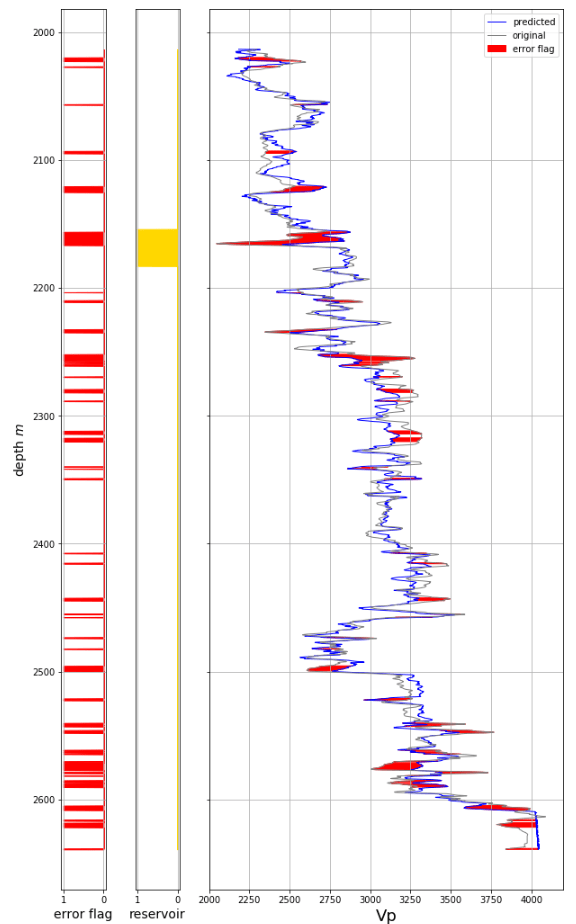Summary statistics can also be calculated by stratigraphic unit, as demonstrated in the accompanying Jupyter Notebook.

# Geophysics Python sprint 2018 – day 2 and beyond, part I

In my last post I wrote about what I did on day one of the Geophysics sprint run by Agile Scientific in Santa Ana two weeks ago.

This post and the next one are about the project Volodymyr and I worked on during day two of the sprint, and continued to work on upon our return to Calgary.

We had read a great notebook by Alessandro Amato del Monte (I recommend browsing his Geophysical notes repo) showing how to reconstruct a velocity log from density with optimized alpha and beta parameters for the Inverse Gardner function, found via scipy.curve_fit.

Inspired by that, we set out with a dual goal:

• First, we wanted to adapt Alessandro’s optimization idea so that it would work with Bruges‘ Inverse Gardner
• Second, we wanted to adapt a function from some old work of mine to flag sections of the output velocity log with poor prediction; this would be useful to learn where alpha and beta may need to be tweaked because of changes in the rock lithology or fluid content

I’ll walk you through some of our work. Below are the two functions:

# Alessandro's simple inverse Gardner
def inv_gardner(rho, alpha, beta):
return (rho/alpha)**(1/beta)

# Bruges' inverse Gardner
def inverse_gardner(rho, alpha=310, beta=0.25, fps=False):
"""
Computes Gardner's density prediction from P-wave velocity.
Args:
rho (ndarray): Density in kg/m^3.
alpha (float): The factor, 310 for m/s and 230 for fps.
beta (float): The exponent, usually 0.25.
fps (bool): Set to true for FPS and the equation will use the typical
value for alpha. Overrides value for alpha, so if you want to use
your own alpha, regardless of units, set this to False.
Returns:
ndarray: Vp estimate in m/s.
"""
alpha = 230 if fps else alpha
exponent = 1 / beta
factor = 1 / alpha**exponent
return factor * rho**exponent

They look similarly structured, and take the same arguments. We can test them by passing a single density value and alpha/beta pair.

inv_gardner(2000, 0.39, 0.23)
>>> 1.349846231542594e+16

inverse_gardner(2000, 0.39, 0.23)
>>> 1.3498462315425942e+16

Good. So the next logical step would be to define some model density and velocity data (shamelessly taken from Alessandro’s notebook, except we now use Bruges’ Gardner with S.I. units) and pass the data, and Bruges’ inverse Gardner toscipy.curve_fit to see if it does just work; could it be that simple?

# Make up random velocity and density with Bruges' direct Gardner
vp_test = numpy.linspace(1500, 5500)
rho_test = gardner(vp_test, 310, 0.25)
noise = numpy.random.uniform(0.1, 0.3, vp_test.shape)*1000
rho_test = rho_test + noise

The next block is only slightly different from Alessandro’s notebook. Instead of using all data, we splits both density and velocity into two pairs of arrays: a rho12 and vp2 to optimize foralpha and beta,  a rho1 for calculating “unknown” velocities vp_calc1 further down; the last one, v1, will be used just to show where the real data might have been had we not had to calculate it.

idx = np.arange(len(vp_test))
np.random.seed(3)
spl1 = np.random.randint(0, len(vp_test), 15)
spl2 = np.setxor1d(idx,spl1)
rho1 = rho_test[spl1]
rho2 = rho_test[spl2]
vp1= vp_test[spl1] # this we pretend we do not have
vp2= vp_test[spl2]

Now, as in Alessandro’s notebook, we pass simple inverse Gardner function to scipy.curve_fit to find optimal alpha and beta parameters, and we printalpha and beta.

popt_synt2, pcov2 = scipy.curve_fit(inv_gardner,rho2, vp2)
print (popt_synt2)
>>> [3.31376056e+02 2.51257203e-01]

Those values seem reasonable, but just to be sure let’s calculate vp_calc1 from rho1 and plot everything to be sure.

vp_calc1 = inv_gardner(rho1, *popt_synt2)

# this is to show the fit line
rho_synt_fit=np.linspace(1, 3000, 50)
vp_synt_fit=inv_gardner(rho_synt_fit, *popt_synt2)

plt.figure(figsize=(10, 10))
plt.plot(rho2,vp2,'or', markersize = 10, label = "fitted points")
plt.plot(rho1,vp1,'ob', markersize = 10, alpha = 0.4, label = "calculated points")
plt.plot(rho1,vp1,'ok', markersize = 10, label = "withheld points")
plt.plot(rho_synt_fit, vp_synt_fit, '-r', lw=2,
label='Fit' r'$V_p=(%.2f / \rho)^{1/%.2f}$' %(popt_synt2,
popt_synt2))
plt.xlabel('Density rho [kg/m^3]'), plt.xlim(1800, 3000)
plt.ylabel('Velocty Vp [m/s]'), plt.ylim(1000, 6000)
plt.grid()
plt.legend(loc='upper left')
plt.show()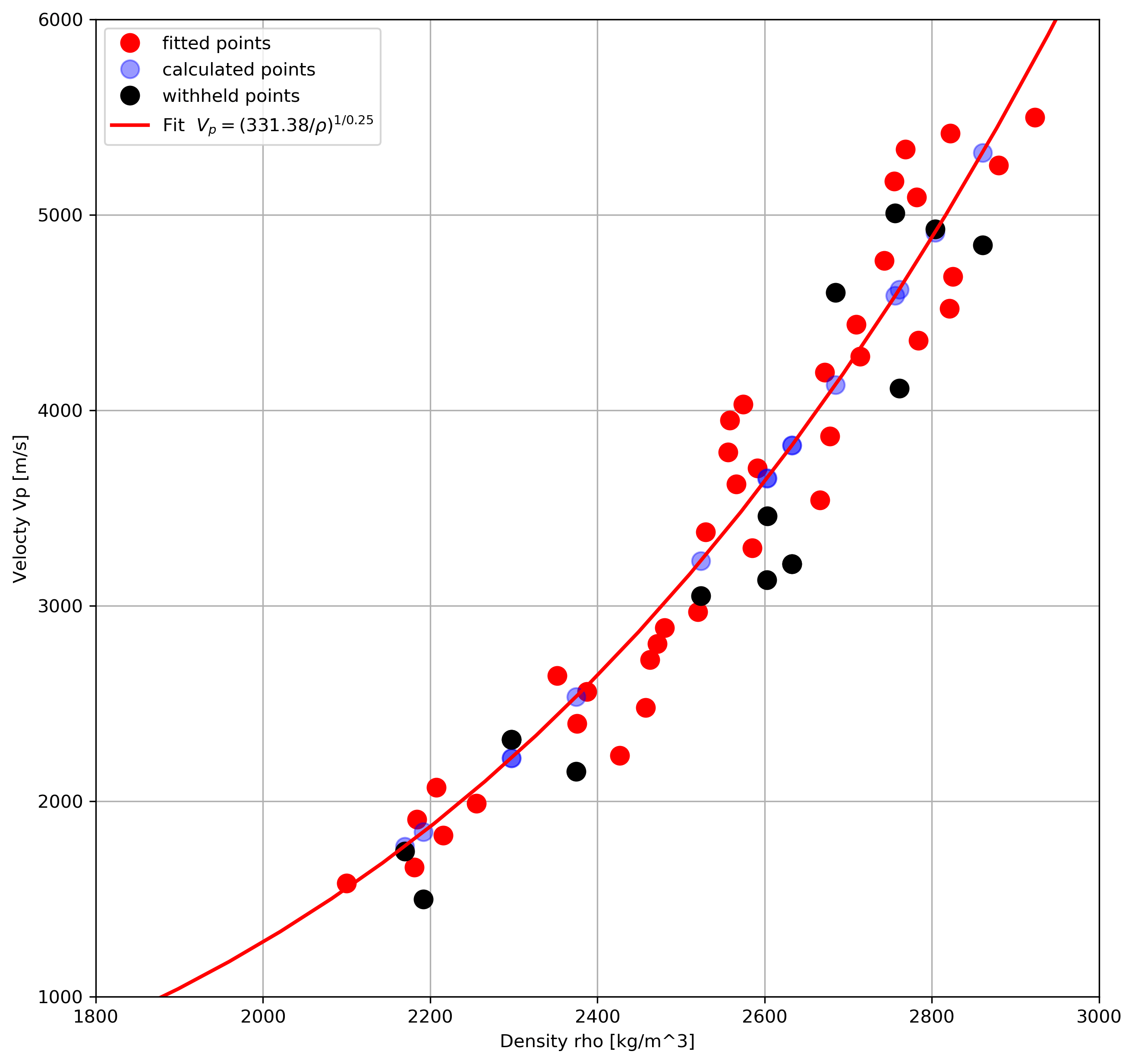That looks great. Let’s now try the same using Bruges’ Inverse Gardner.

popt_synt2, pcov2 = curve_fit(inverse_gardner, rho2, vp2)
print (popt_synt2)
>>> [1.         0.29525991 1.        ]

That is odd, we do not get the same parameters; additionally, there’s this error message:

../scipy/optimize/minpack.py:794:
OptimizeWarning: Covariance of the parameters could not be estimated
category=OptimizeWarning)

One possible explanation is that although both inv_gardner and inverse_gardner take three parameters, perhaps scipy.curve_fit does not know to expect it because in the latter alpha and betaare pre-assigned.

The workaround for this was to write a wrapper function to ‘map’ between the call signature of scipy.curve_fit and that of inverse_gardner so that it would be ‘communicated’ to the former explicitly.

def optimize_inverse_gardner(rho, alpha, beta):
return inverse_gardner(rho, alpha=alpha, beta=beta)

popt_synt2, pcov2 = scipy.curve_fit(optimize_inverse_gardner,
rho2, vp2)
print (popt_synt2)
>>> [3.31376060e+02 2.51257202e-01]

Which is the result we wanted.

In the next post we will apply this to some real data and show how to flag areas of poorer results.

# Geophysics Python sprint 2018 – day 1

Last weekend I went to California to attend my first ever Python sprint, which was organized at MAZ Café con leche (Santa Ana) by Agile Scientific.

For me this event was a success in many respects. First of all, I wanted to spend some dedicated time working on an open source project, rather than chipping away at it once in a while. Also, participating in a project that was not my own seemed like a good way to challenge myself, by pushing me out of a zone of comfort. Finally, this was an opportunity to engage with other members of the Software Underground Slack team, some of which (for example Jesper Dramsch and Brendon Hall) I’ve known for some time but actually never met in person.

Please read about the Sprint in general on Matt Hall‘s blog post, Café con leche. My post is a short summary of what I did on the first day.

After a tasty breakfast, and at least a good hour of socializing, I sat at a table with three other people interested in working on Bruges (Agile’s Python library for Geophysics) : Jesper Dramsch, Adriana Gordon and Volodymyr Vragov.

As I tweeted that evening, we had a light-hearted start, but then we set to work.While Adriana and Jesper tackled Bruges’ documentation, which was sorely needed, Volodymyr spent some hours on example notebooks from in-Bruges (a tour of Bruges), which needed fixing, and also on setting up our joint project for day 2 (more in the next post). For my part, I  put together a tutorial notebooks on how to use Bruges’ functions on wireline logs stored in a Pandas DataFrame. According to Matt, this is requested quite often, so it seemed like a good choice.

Let’s say that a number of wells are stored in a DataFrame with both a depth column, and a well name column, in addition to log curves.

The logic for operating on logs individually is this:
Split the wells in the DataFrame using groupby, then
for each well
for each of the logs of interest
do something using one of Bruges’ functions (for example apply a rolling mean)

The code to do that is surprisingly simple, once you’ve figure it out (I myself struggle often, and not little with Pandas at the outset of new projects).

One has to first create a list with the logs of interest, like so:

logs = ['GR', 'RHOB']

then define the length of the window for the rolling operation:

window = 9

finally, the logic above is applied as:

wells_sm=pd.DataFrame()

grouped=wells['well'].unique()

for well in grouped:
new_df=pd.DataFrame()
sm=br.filters.mean(np.array(wells[log][wells['well']==well]),
window)
new_df[str(log) + '_sm']=sm
wells_sm=pd.concat([wells_sm, new_df])


where wells_sm is a temporary DataFrame for the filtered logs, which can be added back to the original DataFrame with:

wells_filtered = (np.concatenate((wells.values,
wells_sm.values), axis=1))
cols = list(wells) + list(wells_sm)
wells_filtered_df = pd.DataFrame(wells_filtered, columns=cols)

You can work through the full example in the notebook.

# Machine Learning in Python: classification using Support Vector Machines and Scikit-learn

This post is a short extract, with minor modifications,  from my recently released article on the check the CSEG Recorder Machine Learning in Geoscience V: Introduction to Classification with SVMs.

### Understanding classification with Support Vector Machines

Support Vector Machines are a popular type of algorithm used in classification, which is the process of  “…identifying to which of a set of categories (sub-populations) a new observation belongs (source: Wikipedia).

In classification, the output variable is a category, for example ‘sand’, or ‘shale’, and the main task of the process is the creation of a dividing boundary between the classes. This boundary will be a line in a bi-dimensional space (only two features used to classify), a surface in a three dimensional space (three features), and a hyper-plane in a higher- dimensional space. In this article I will use interchangeably the terms hyper-plane, boundary, and decision surface.

Defining the boundary may sound like a simple task, especially with two features (a bidimensional scatterplot), but it underlines the important concept of generalization, as pointed out by Jake VanderPlas in his Introduction to Scikit-Learn, because ”… in drawing this separating line, we have learned a model which can generalize to new data: if you were to drop a new point onto the plane which is unlabeled, this algorithm could now predict…” the class it belongs to.

Let’s use a toy classification problem to understand in more detail how in practice SVMs achieve the class separation and find the hyperplane. In the figure below I show an idealized version (with far fewer points) of a Vp/Vs ratio versus P-impedance crossplot from Amato del Monte (2017, Seismic rock physics, tutorial on The Leading Edge).  I’ve added three possible boundaries (dashed lines) separating the two classes.

Each boundary is valid, but are they equally good? Well, for the SVM classifier, they are not because the classifier looks for the boundary with the largest distance from the nearest point in either of the classes.

These points, called Support Vectors, are the most representative of each class, and typically the most difficult to classify. They are also the only ones that matter; if a Support Vector is moved, the boundary will also move. However, if any other point is moved, provided that it is not moved into the margin or across the boundary, it would have no effect on the boundary. This makes SVM classifiers insensitive to outliers (points very far away from the rest of the points in their class and from the boundary) and also less memory intensive than other classifiers (for example, the perceptron). The process of finding this boundary is referred to as “maximizing the margin”, where the margin is a corridor with no data points between the boundary and the support vectors. The larger this buffer, the lower the generalization error; conversely, small margins are almost invariably associated with over-fitting. We will see more on this in a subsequent section.

So, to go back to the question, which of the three proposed boundaries is the best one (and by “best” I am referring to the one that will generalize better to unseen data)? Based on what we’ve learned so far, it would have to be the green boundary. Indeed, the orange one is so close to its support vectors (the two points circled with orange) that it leaves virtually no margin; the purple boundary is slightly better (the support vectors are the points circled with purple) but its margin is still quite small compared to the green boundary.

Maximizing the margin is the goal of the SVM classifier, and it is a constrained optimization problem. I refer interested readers to Hearst (1998, Support Vector Machines, IEEE Intelligent Systems); however, I will quote a definition from that paper (with reference to Figure 1 and accompanying text) as it yields further understanding: “… the optimal hyper-plane is orthogonal to the shortest line connecting the convex hulls of the two classes, and intersects it half way”.

In the inset in the figure, I zoomed closer to the 4 points near the green boundary; I’ve also drawn the convex hulls for the classes, the margin, and the shortest orthogonal line, which is bisected by the hyper-plane. I have selected (by hand) the best hyper-plane already (the green one), but if you can imagine rotating a line to span all possible orientations in the empty space close to the two classes without intersecting either of the hulls and find the one with the largest margin, you’ve just done quadratic optimization in your head. Moreover, you’ve turned a crossplot into a decision surface (quoted from Sebastian Thrun,  Intro to Machine Learning, Udacity 120 course).

If you are interested in learning more about Support Vector Machines in an intuitive way, and then how to try classification in practice (using Python and the Scikit-learn library), read the full article here, check the GitHub repo, then read How good is what? (blog post by Evan Bianco of Agile Scientific) for an example and DIY evaluation of  classifier performance.